# Working Capital Management – Financial Management MCQ

Working Capital Management – CS Executive Financial and Strategic Management MCQ Questions with Answers you can quickly revise the concepts.

## Working Capital Management – Financial Management MCQ

Question 1.
Working capital is also known
(A) Operation capital
(B) Operating capital
(C) Current assets capital
(D) Capital relating to main projects of the company
(B) Operating capital

Question 2.
A positive working capital means that –
(A) the company is able to pay-off its long-term liabilities.
(B) the company is able to select profitable projects.
(C) the company is unable to meet its short-term liabilities.
(D) the company is able to pay-off its short-term liabilities.
(D) the company is able to pay-off its short-term liabilities.

Question 3.
Working capital =………….
(A) Core current assets less current liabilities
(B) Core current assets less core current liabilities
(C) Liquid assets less current liabilities
(D) Current assets less current liabilities
(D) Current assets less current liabilities

Question 4.
Other things remaining constant, if the debtors increases as compared to last year it means –
(A) Company has poor credit policy
(B) Company has positive working capital
(C) Company has negative working capital
(D) Company has no working capital
(B) Company has positive working capital

Question 5.
Which of the following will be considered while calculating working capital?
(2) Stock of WIP
(3) Short Term Investments
(4) Perpetual inventory policy
Select the correct answer from the options given below.
(A) (2) & (3)
(B) (1) & (3)
(C) (1), (2) & (3)
(D) All of the above except (4)
(D) All of the above except (4)

Question 6.
Contingencies are – ………..
(A) Added to gross working capital
(B) Deducted from gross working capital
(C) Contingencies are not considered in financial management; it is considered in accounts only
(D) None of the above
(A) Added to gross working capital

Question 7.
For reducing and controlling working capital requirement which of the following step is required to be taken –
(A) Increase in manufacturing cycle
(B) Increase of credit period allowed by creditors to the extent that do not affect the production.
(C) Increase in credit period given to customers
(D) All of the above
(B) Increase of credit period allowed by creditors to the extent that do not affect the production.

Question 8.
Working capital is a highly effective barometer of a company’s efficiency and effectiveness.
(A) operational and servicing
(B) long term
(C) operational and financial
(D) positive and negative
(C) operational and financial

Question 9.
Statement I:
Maintaining adequate working capital is not just important in the short-term. Sufficient liquidity must be maintained in order to ensure the survival of the business in the long-term as well.
Statement II:
Even a profitable business may fail if it does not have adequate cash flow to meet its liabilities as they fall due.
Select the correct answer from the options given below.
(A) Statement I is correct while Statement II is incorrect.
(B) Statement II is correct while Statement I is incorrect.
(C) Both Statement I and Statement II are correct.
(D) Both Statement I and Statement II are incorrect.
(C) Both Statement I and Statement II are correct.

Question 10.
While calculating working capital based on cash cost –
(A) Depreciation is ignored
(B) Non-cash items are not considered
(C) Debtors are calculated on the basis of cost of goods sold and not on sale price
(D) All of the above
(D) All of the above

Question 11.
A negative working capital means that -………..
(A) the company has no current assets at all
(B) the company currently is unable to meet its short-term liabilities
(C) the company has negative earnings before interest and tax
(D) the company currently is able to meet its short-term liabilities
(B) the company currently is unable to meet its short-term liabilities

Question 12.
Which of the following analyzes the accounts receivable, inventory and accounts payable cycles in terms of number of days?
(A) Operation cycle
(B) Current asset cycle
(C) Operating cycle
(C) Operating cycle

Question 13.
Which of the following method is not used for calculating working capital cycle?
(A) Percentage of sales method
(B) Regression analysis method
(C) Operating cycle approach
(D) Trial and error method
(D) Trial and error method

Question 14.
Which of the following is correct formula to calculate WIP Conversion Period?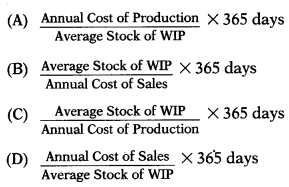(C)

Question 15.
Initial Working Capital -…………
(A) supplies the funds necessary to meet the current working expenses.
(B) is used to raise the volume of production by improvement or extension of machinery.
(C) is required at the time of the commencement of business
(D) represents the amount utilized at the time of contingencies.
(C) is required at the time of the commencement of business

Question 16.
Which of the following is determinant of working capital?
(1) Nature and size of business
(2) Manufacturing cycle
(3) Credit policy
(4) Production policy
Select the correct answer from the options given below.
(A) (1) only
(B) (1) and (2) only
(C) (1), (2) and (3) only
(D) (1), (2), (3) and (4)
(D) (1), (2), (3) and (4)

Question 17.
Which of the following statement is correct?
(A) A desire to maintain an established dividend policy may affect the volume of working capital.
(B) Changes in working capital may bring about an adjustment of dividend policy.
(C) Payment of dividend may reduce cash in current assets considerably which in turn may reduce the available working capital for the company.
(D) All of the above
(D) All of the above

Question 18.
Regular Working Capital –
(A) supplies the funds necessary to meet the current working expenses ie. for purchasing raw material and supplies, payment of wages, salaries and other sundry expenses.
(B) refers to the firm’s investment in current assets.
(C) is amount over and above the permanent level of working capital.
(D) refers to the difference between current asset and Current liabilities.
(A) supplies the funds necessary to meet the current working expenses ie. for purchasing raw material and supplies, payment of wages, salaries and other sundry expenses.

Question 19.
Which of the following is correct formula to calculate working capital leverage?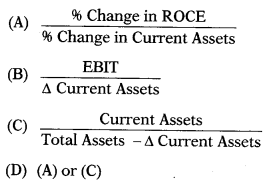(D)

Question 20.
One of the important objective(s) of working capital management is/are –
(A) To maintain the optimum levels of investment in current assets.
(B) To reduce the levels of current liabilities.
(C) Improve the return on capital employed.
(D) All of the above
(D) All of the above

Question 21.
Fluctuating Working Capital is also called as —
(A) Reserve Margin Working Capital
(B) Temporary Working Capital
(C) Permanent Working Capital
(D) Variable working capital
(D) Variable working capital

Question 22.
Operating cycle is also called as –
(A) Working cycle
(C) Current asset cycle
(D) Working capital cycle
(D) Working capital cycle

Question 23.
For reducing and controlling working capital requirement which of the following step is required to be taken –
(i) Reduction of manufacturing cycle.
(ii) Increase of credit period allowed by creditors to the extent that do not affect the production.
(iii) Reduction in credit period given to customers.
Select the correct answer from the options
given below
(A) (i) &(iii)
(B) (ii) & (iii)
(C) (i) & (ii)
(D) All of the above
(D) All of the above

Question 24.
Capital which is needed to meet the seasonal requirements of the business –
(A) Gross Working Capital
(B) Reserve Margin Working Capital
(C) Net working capital
(D) Fluctuating Working Capital
(D) Fluctuating Working Capital

Question 25.
A higher current assets/fixed assets ratio indicates –
(A) Hedging Approach
(B) Conservative Approach
(C) Matching/hedging Approach
(D) Aggressive Approach
(B) Conservative Approach

Question 26.
Aggressive approach covers those policies –
(A) where the firm relies heavily on short term bank finance.
(B) seeks to increase dependence on long term financing.
(C) Both (A) and (B)
(D) Neither (A) nor (B)
(A) where the firm relies heavily on short term bank finance.

Question 27.
Gross working capital refers to –
(A) the amount utilized at the time of contingencies.
(B) the firm’s investment in current assets.
(C) the capital which is required at the time of the commencement of business.
(D) the working capital which is necessary on a continuous and uninterrupted basis.
(B) the firm’s investment in current assets.

Question 28.
A conservative policy implies –
(A) greater liquidity and lower risk
(B) greater risk and lower liquidity
(C) negligible risk
(D) no risk at all with low liquidity
(A) greater liquidity and lower risk

Question 29.
If a firm has insufficient working capital and tries to increase sales, it can easily over-stretch the financial resources of the business. This is called –
(A) Overrating
(C) Overcoming
(D) Overtone

Question 30.
Which of the following represents the amount utilized at the time of contingencies?
(A) Reserve Working Capital
(B) Net working capital
(C) Extra working capital
(D) Fixed working capital
(A) Reserve Working Capital

Question 31.
Permanent Working Capital is also known as –
(A) Fixed working capital
(B) Temporary working capital
(C) Long term funds
(D) Gross margin working capital
(A) Fixed working capital

Question 32.
A lower current assets/fixed assets ratio means –
(A) Matching/hedging Approach
(B) Aggressive current assets policy
(C) Riskier current assets policy
(D) Conservative current assets policy
(B) Aggressive current assets policy

Question 33.
Any amount over and above the permanent level of working capital is known as working capital.
(A) Temporary
(B) Fluctuating
(C) Variable
(D) All of the above
(D) All of the above

Question 34.
Current assets are those assets –
(A) Which can be sold by the companies.
(B) Which are less important from production angle.
(C) Which are held by the companies to pay-off current liabilities.
(D) Which are converted in to cash within a period of one year.
(D) Which are converted in to cash within a period of one year.

Question 35.
Current assets are usually financed through –
(A) equity capital, preference capital, debentures, bonds and long term bank loans.
(B) (A) and (C)
(C) mode of overdraft, cash credit, public deposits etc.
(D) money market instrument and long term securities.
(C) mode of overdraft, cash credit, public deposits etc.

Question 36.
To carry on a business, a certain minimum level of working capital is necessary on a continuous and uninterrupted basis. This requirement is referred to as –
(A) Permanent working capital
(B) Long term working capital
(C) Fixed working capital
(D) Both (A) and (C)
(D) Both (A) and (C)

Question 37.
Which of the following is/are method of maximum permissible bank finance as recommended by the Tandon Committee?
(A) 75% of (Current Assets – Current Liabilities)
(B) 50% of (Current Assets – Current Liabilities)
(C) 75%of(CoreCurrentAssets-Current Liabilities)
(D) 50% of (Core Current Assets-Current Liabilities)
(A) 75% of (Current Assets – Current Liabilities)

Question 38.
………… varies inversely with profitability.
(A) Liquidity
(B) Risk
(C) Gross profit
(D) None of the above
(A) Liquidity

Question 39.
………….. refers to the difference between current asset and current liabilities.
(A) Differential working capital
(B) Net working capital
(C) Operation working capital
(D) None of the above
(B) Net working capital

Question 40.
Permanent working capital -…………
(A) varies with seasonal needs.
(B) includes fixed assets.
(C) is the amount of current assets required to meet a firm’s long-term minimum needs.
(D) includes accounts payable.
(C) is the amount of current assets required to meet a firm’s long-term minimum needs.

Question 41.
Financing a long-lived asset with short-term financing would be
(A) an example of “moderate risk-moderate (potential) profitability” asset financing.
(B) an example of “low risk-low (potential) profitability” asset financing.
(C) an example of “high risk – high (potential) profitability” asset financing.
(D) an example of the “hedging approach” to financing.
(C) an example of “high risk – high (potential) profitability” asset financing.

Question 42.
Hard core working capital is also known as –
(A) Hard current assets
(B) Core current assets
(C) Core current liabilities
(D) Hard current liabilities
(B) Core current assets

Question 43.
An aggressive policy indicates –
(A) higher liquidity and poor risk
(B) higher risk and poor liquidity
(C) higher risk and higher liquidity
(D) lower risk with lower liquidity
(B) higher risk and poor liquidity

Question 44.
Tandon Committee Report on Working Capital relates to norms for ………….
(A) inventory and receivables
(B) gross profit and inventory
(C) receivable, gross profit and inventory
(D) net profit and receivables
(A) inventory and receivables

Question 45.
In deciding the appropriate level of current assets for the firm, management is confronted with –
(A) Trade-off between profitability and risk.
(B) Trade-off between liquidity and marketability.
(C) Trade-off between equity and debt.
(D) Trade-off between short-term versus long-term borrowing.
(A) Trade-off between profitability and risk.

Question 46.
Which of the following is not correct with matching strategy?
(A) All assets should be financed with permanent long term capital.
(B) Temporary current assets should be financed with temporary working capital.
(C) Long term assets should be financed from long term capital.
(D) Permanent current assets should be financed with permanent working capital.
(A) All assets should be financed with permanent long term capital.

Question 47.
Paucity of working capital may lead to a situation where –
(A) the firm may not be able to its long term finance
(B) the firm may not be able to meet its liabilities
(C) the firm may not be able to achieve its sale target
(D) the firm may taken some different project with low internal rate of return.
(B) the firm may not be able to meet its liabilities

Question 48.
Which of the following is/are method of maximum permissible bank finance as recommended by the Tandon Committee?
(A) [50% of (Current Assets – Core Current Assets)] – Current Liabilities
(B) [75% of (Core Current Assets – Current Assets)] – Current Liabilities
(C) [75% of (Current Assets – Core Current Assets)] – Current Liabilities
(D) [80% of (Current Assets – Core Current Assets)] – Current Liabilities
(C) [75% of (Current Assets – Core Current Assets)] – Current Liabilities

Question 49.
What is difference between current ratio and quick ratio?
(A) The current ratio includes inventory and quick ratio does not.
(B) The current ratio does not include inventory and quick ratio does.
(C) The current ratio includes physical capital and quick ratio does not.
(D) The current ratio does not include physical capital and quick ratio does.
(A) The current ratio includes inventory and quick ratio does not.

Question 50.
It is understood that a current ratio of ……….. for a manufacturing firm implies that the firm has an optimum amount of working capital.
(A) 1 (one)
(B) 2 (two)
(C) 3 (three)
(D) 2.5 (two and half)
(B) 2 (two)

Question 51.
Which of the following is relevant while planning for working capital requirement?
(A) Identify the cash balance which allows for the business to meet day to day expenses, but reduces cash holding costs.
(B) Identify the level of inventory which allows for uninterrupted production.
(C) Identify the appropriate credit policy.
(D) All of the above
(D) All of the above

Question 52.
A conservative policy means –
(A) lower return and risk.
(B) higher return and risk.
(C) lower return but very high risk.
(D) higher return and higher risk.
(A) lower return and risk.

Question 53.
An aggressive policy produces –
(A) higher return and risk
(B) lower return and risk
(C) lower return and very low risk
(D) none of the above
(A) higher return and risk

Question 54.
Accounts receivable are analyzed by –
(A) the average number of days it takes to make sale.
(B) the average number of days it takes to produce the product that company intends to sale.
(C) the average number of days it takes to collect an account.
(D) Any of the above
(C) the average number of days it takes to collect an account.

Question 55.
Which of the following would not be financed from working capital?
(A) Cash float.
(B) Accounts receivable.
(C) Credit sales.
(D) A new personal computer for the office
(D) A new personal computer for the office

Question 56.
Which of the following is/are method of maximum permissible bank finance as recommended by the Tandon Committee?
(A) 60% of Current Assets – Current Liabilities
(B) 7596 of Current Assets – Current Liabilities
(C) 5096 of Current Assets – Current Liabilities
(D) 4096 of Current Assets – Current Liabilities
(B) 7596 of Current Assets – Current Liabilities

Question 57.
Net working capital refers to –
(A) total assets minus fixed assets.
(B) current assets minus current liabilities.
(C) current assets minus inventories.
(D) current assets.
(B) current assets minus current liabilities.

Question 58.
Which of the following is correct formula to calculate debtor’s collection period?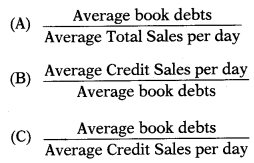(C)

Question 59.
Which of the following working capital strategies is the most aggressive?
(A) Making greater use of short term finance and maximizing net short term asset.
(B) Making greater use of long term finance and minimizing net short term asset.
(C) Making greater use of short term finance and minimizing net short term asset.
(D) Making greater use of long term finance and maximizing net short term asset.
(C) Making greater use of short term finance and minimizing net short term asset.

Question 60.
Working capital is also known as –
(A) Current capital or circulating capital
(B) Work-in-progress capital
(C) Day-to-day capital
(A) Current capital or circulating capital

Question 61.
Inventory is listed as a part of current assets. Stock or inventory in an organization means
(A) Goods that are readily available for sale
(B) All the assets available for sale
(C) All the raw material, Semi-finished and the finished goods in the organization
(D) Goods that can be sold within a short span of time
(C) All the raw material, Semi-finished and the finished goods in the organization

Question 62.
What are the aspects of working capital management?
I. Inventory management
II. Receivable management
III. Cash management
Select the correct answer from the options given below.
(A) I
(B) II
(C) III
(D) All of the above
(D) All of the above

Question 63.
Working Capital Turnover measures the relationship of Working Capital with:
(A) Fixed Assets
(B) Sales
(C) Purchases
(D) Stock
(B) Sales

Question 64.
Accounts payable are analyzed by the –
(A) average number of days it takes to pay a supplier fixed assets.
(B) average number of days it takes to pay a supplier invoice.
(C) average number of days it takes to pay a salary of employee.
(D) Any of the above
(B) average number of days it takes to pay a supplier invoice.

Question 65.
Which of the following is correct formula to calculate credit period availed?(B)

Question 66.
In Current Ratio. Current Assets are compared with:
(A) Current Profit
(B) Current Liabilities
(C) Fixed Assets
(D) Equity Share Capital
(B) Current Liabilities

Question 67.
There is deterioration in the management of working capital of XYZ Ltd. What does it refer to?
(A) That the Capital Employed has reduced
(B) That the Profitability has gone up
(C) That debtors collection period has increased
(D) That Sales has decreased.
(C) That debtors collection period has increased

Question 68.
…………… refers to the length of time allowed by a firm for its customers to make payment for their purchases.
(A) Holding period
(B) Pay-back period
(C) Average collection period
(D) Credit period
(D) Credit period

Question 69.
Working capital management is primarily concerned with the management and financing of:
(A) Cash & inventory
(B) Current assets & current liabilities
(C) Current assets
(D) Receivables and payables
(B) Current assets & current liabilities

Question 70.
Operating cycles period equals:
(A) Collection period+Inventory holding period – Creditor Payment Period
(B) Collection period-Inventory holding period + Creditor Payment Period
(C) Creditor Payment Period-(-Inventory holding period – Collection period
(D) Any of the above
(A) Collection period+Inventory holding period – Creditor Payment Period

Question 71.
Which of the following is not a metric to use for measuring the length of the cash cycle?
(A) Acid test days
(B) Accounts receivable days
(C) Accounts payable days
(D) Inventory days
(A) Acid test days

Question 72.
Decrease in current assets means –
(A) Increase in working capital
(B) Decrease in inventories
(C) Decrease in working capital
(D) Increase in accounts payable days.
(C) Decrease in working capital

Question 73.
Which of the following is not considered while calculating accounts receivable period?
(A) Bills receivable
(B) Cash sales
(C) Debtors
(D) Credit sales
(B) Cash sales

Question 74.
Which of the following will not be considered while calculating working capital on cash cost basis?
(A) Depreciation
(B) Prepaid expenses
(C) Raw material consumed
(D) Bills receivable
(A) Depreciation

Question 75.
Which of the following assets is not a quick current asset?
(A) Short term bills receivables
(B) Cash
(C) Stock
(D) Debtors less provision for bad and doubtful debts
(C) Stock

Question 76.
………… is also known as working capital ratio.
(A) Current ratio
(B) Quick ratio
(C) Liquid ratio
(D) Working capital turnover ratio
(A) Current ratio

Question 77.
What does the accounts receivable turnover ratio tell us?
(A) How often account receivable received
(B) How many time account receivable is collected
(C) Account receivable balance at the end of the period
(D) Bad debt balance at the year end
(B) How many time account receivable is collected

Question 78.
The best ratio to evaluate short-term liquidity is:
(A) Working capital turnover ratio
(B) Current ratio
(C) Creditors velocity
(D) All of the above
(B) Current ratio

Question 79.
Which best describes the gross margin ratio?
(A) Leverage ratio
(B) Liquidity ratio
(C) Coverage ratio
(D) Profitability ratio
(D) Profitability ratio

Question 80.
Inventory turnover ratio evaluates:
(A) Company’s ability to move inventory
(C) Both (A) & (B)
(D) None of the above
(C) Both (A) & (B)

Question 81.
All of the following statements are true regarding ratios that measure a company’s ability to pay current liabilities except
(A) Working Capital = Current Assets – Current Liabilities
(B) A higher current ratio is always preferred to a lower current ratio.
(C) Inventory and prepaid expense are included in the numerator of the current ratio, but not in the numerator of the acid-test ratio.
(D) In most industries, a current ratio of 2.0 is considered adequate.
(B) A higher current ratio is always preferred to a lower current ratio.

Question 82.
Which of the following would NOT improve the current ratio?
(A) Borrow short term to finance additional fixed assets.
(B) Issue long-term debt to buy inventory
(C) Sell common stock to reduce current liabilities
(D) Sell fixed assets to reduce accounts payable
(A) Borrow short term to finance additional fixed assets.

Question 83.
If the trend of the current ratio is increasing, while the trend of the acid-test ratio is decreasing over a period of time, this could be a warning that the firm is:
(A) Depleting its inventories
(B) Having trouble collecting its receivables
(C) Purchasing too much treasury stock
(D) Carrying excess inventories
(D) Carrying excess inventories

Question 84.
What relationship exists between the average collection period and accounts receivable turnover?
(A) As average collection period increases (decreases) the accounts receivable turnover decreases (increases)
(B) There is a direct and proportional relationship
(C) Both ratios are expressed in number of days
(D) Both ratios are expressed in number of times receivables are collected per year
(A) As average collection period increases (decreases) the accounts receivable turnover decreases (increases)

Question 85.
Which of the following statements is most correct?
(A) If a company increases its current liabilities by ₹ 1,000 and simultaneously increases its inventories by ₹ 1,000, its current ratio must rise.
(B) If a company increases its current liabilities by ₹ 1,000 and simultaneously increases its inventories by ₹ 1,000, its quick ratio must fall.
(C) A company’s quick ratio never exceed its current ratio.
(D) (B) & (C) is correct.
(D) (B) & (C) is correct.

Question 86.
Purchase of stock for cash will current ratio.
(A) Reduce
(B) Improve
(C) Not change
(D) Can’t say
(C) Not change

Question 87.
Explain the important ratio that would be used in following situation:
A bank is approached by a company for a loan of ₹ 50 lakh for working capital purposes.
(A) Capital Structure /Leverage Ratios
(B) Profitability Ratios
(C) Liquidity Ratios
(D) Activity Ratios
(C) Liquidity Ratios

Question 88.
Instead of “Annual Factory Cost” WIP Conversion Period can be calculated taking “ ……….” as base.
(A) Annual Sales
(B) Cost of Production
(C) Cost of Goods Sold
(D) Cost of Sales
(B) Cost of Production

Question 89.
If annual sales figure is not available then which of the following figure will be taken as base for calculation of debtors?
(A) Factory Cost
(B) Cost of Production
(C) Cost of Goods Sold
(D) Cost of Sales
(B) Cost of Production

Question 90.
If current assets increases by 20% and current liabilities decreases by 20% as compared to last year figures then –
(A) Working capital decreases as compared to last year
(B) Working capital increases as compared to last year
(C) There will be no change in working capital
(D) Without figures it is impossible to tell whether working capital will increase or decrease
(B) Working capital increases as compared to last year

Question 91.
If raw material consumed is ₹ 8,42,000; cost of production is ₹ 14,25,000; Stock of raw material & WIP is ₹ 1,24,000 and 1,72,000 respectively then Raw Material Conversion period will be –
Note: 1 Year = 365 days
(A) 54 days
(B) 18 days
(C) 29 days
(D) 49 days
(A) 54 days
Raw material conversion periodQuestion 92.
Calculate the working capital from the following data:
Particulars — X
Raw Material Stock — 11,70,000
WIP Stock — 9,58,750
Finished Goods Stock — 26,65,000
Debtors — 55,12,000
Cash & Bank — 6,00,000
Creditors — 17,55,000
Outstanding expenses — 14,95,000
(A) 76,75,550
(B) 76,55,750
(C) 75,65,750
(D) 77,55,650
(B) 76,55,750
Working capital = 11,70,000 + 9,58150 + 26,65,000 + 55,12,000 + 6,00,000 – 17,55,000 – 14,95,000 = 76,55,750

Question 93.
Annual credit sales Cash sales Debtors Bills receivable Finished goods Collection Period = ?
Note: 1 Year = 360 days
(A) 29 days
(B) 30 days
(C) 49 days
(D) Data given is not sufficient
(C) 49 days
Debtors Collection Period: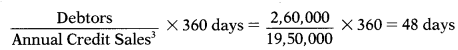Question 94.
Financial statement of A Ltd. shows the following data:
Opening stock ₹ 1,75,000, Total purchase ₹ 10,75,000 including cash purchase ₹ 1,75,000, total sales ₹ 15,00,000 out of which 20% are on cash basis. Closing stock is ₹ 1,50,000. Stock turnover ratio = ?
(A) 7.67
(B) 6.77
(C) 7.76
(D) 7.66
(B) 6.77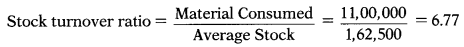Question 95.
WIP Conversion Period =18 days
Raw Material Consumed = ₹ 8,42,000
Stock of WIP = ₹ 72,000
Cost of Production = ?
(A) ₹ 14,00,000
(B) ₹ 22,67,000
(C) ₹ 5,83,000
(D) ₹ 14,60,000
(D) ₹ 14,60,000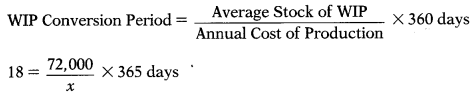x = Annual Cost of Production 14,60,000

Question 96.
A Ltd. financial statement shows the following data:
Equity ₹ 5,67,500, Reserve & surplus ₹ 3,87,850, total debt ₹ 5,88,778 out of which ₹ 2,88,778 are long term debt, fixed assets are ₹ 11,44,128.
Current Ratio = ?
(A) 2.48
(B) 1.92
(C) 3.68
(D) 1.33
(D) 1.33
Current liabilities = 5,88,778 – 2,88,778 = 3,00,000
Total assets = 5,67,500 + 3,87,850 + 5,88,778 = 15,44,128
Current assets = 15,44,128 – 11,44,128 = 4,00,000
Current ratio =$$\frac{4,00,000}{3,00,000}=1.33$$

Question 97.
Total sales of OLX Ltd. are ₹ 31,248 out of which 25% are cash sales. Closing balance of debtors are ₹ 9,468. Debtors collection period = ?
Note: 1 Year — 365 days
(A) 4.2 months
(B) 157 days
(C) 148 days
(D) 4.43 month
(C) 148 daysQuestion 98.
Raw material consumption= ₹ 6,48,000 Raw material purchase = ₹ 8,42,000 Annual cost of production = ₹ 14,42,000 Creditors = ₹ 75,000
Bills payable = ₹ 25,000 Creditors Payment Period = ?
Note: 1 Year = 360 days
(A) 34 days
(B) 43 days
(C) 40 days
(D) 39 days
(B) 43 days
Creditors Payment Period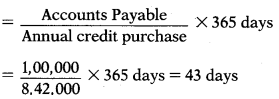Question 99.
The following information is available for your calculation.Level of activity 1.56,000 units
Raw materials are in stock on average two month and Materials are in process, on average half month.
Calculate value of ‘Raw Material Stock’ for working capital purpose.
(A) ₹ 26,65,000
(B) ₹ 23,40,000
(C) ₹ 6,45,250
(D) ₹ 9,58,750
(B) ₹ 23,40,000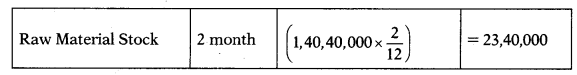Question 100.
From the following data calculate finished goods conversion period for the years 2019 & 2020.Note: 1 Year = 360 days
(A) 29 days & 39 days
(B) 39 days & 29 days
(C) 25 days & 35 days
(D) 35 days & 25 days
(A) 29 days & 39 daysQuestion 101.
Maximum permissible bank finance as per first method of Tandon Committee norms was ₹ 57,41,813 while current liabilities are reported at 32,50,000. Current assets = ?
(A) ₹ 1,09,05,750
(B) ₹ 81,79,313
(C) ₹ 1,09,07,550
(D) ₹ 1,05,09,750
(A) ₹ 1,09,05,750
Maximum permissible bank finance as per first method = 75% of
(Current Assets – Current Liabilities)
57,41,813 = 0.75 × (x – 32,50,000)
57,41,813 = 0.75x – 24,37,500
0.75x = 81,79,313
x = Current Assets = 1,09,05,750

Question 102.
Current assets of Z Ltd. are ₹ 3,70,000 which includes stock ₹ 1,00,000 and prepaid expense ₹ 70,000. Its current liability are ₹ 1,60,000 which includes provision for tax ₹ 60,000.
Liquid Ratio = ?
(A) 1.25
(B) 1.52
(C) 1.22
(D) 0.95
(A) 1.25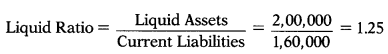Question 103.
Following information is provided by the DPS Ltd. for the year ending 31st March 2019.
Raw material storage period — 55 days
WW conversion period — 18 days
Finished goods storage period — 22 days
Debt collection period — 45 days
Creditor’s payment period — 60 days
Annual operating cost including depreciation of ₹ 2,10,000 was ₹ 21,00,000.
[1 Year =360 days]
You are required to calculate working capital on cash cost basis.
(A) ₹ 4,20,000
(B) ₹ 4,66,667
(C) ₹ 7,35,000
(D) ₹ 8,16,667
(A) ₹ 4,20,000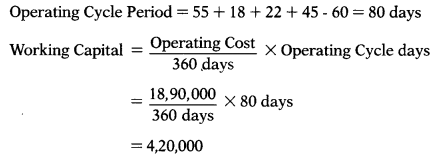Question 104.
KT Ltd. opening stock was ₹ 2,50,000 and closing stock was ₹ 3,75,000. Sales during the year was ₹ 13,00,000 and gross profit ratio was 25% on sales. Average accounts payable are ₹ 80,000. Creditors Turnover Ratio = ?
(A) 13.75
(B) 14.33
(C) 13.33
(D) 14.44
(A) 13.75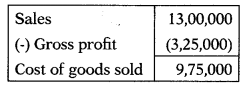2,50,000 + Cost of production/purchase – 3,75,000 = 9,75,000
Cost of production/purchase = 11,00,000
Creditors Turnover Ratio =$$\frac{11,00,000}{80,000}$$ = 13.75

Question 105.
Raw material conversion period is 36 days. Raw material consumed and cost of goods sold in the year is ₹ 1,80,000 & ₹ 2,16,000 respectively. How much raw material stock will appear in working capital statement?
Note: 1 Year = 360 days
(A) ₹ 18,000
(B) ₹ 20,000
(C) ₹ 21,600
(D) ₹ 19,800
(A) ₹ 18,000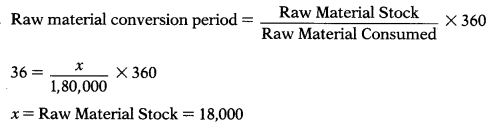Question 106.
Creditors payment period = 60 days Material consumed = ₹ 1,20,000 Material purchased in cash = ₹ 10,000 Material purchased on credit = ₹ 90,000 Creditors that will appear in balance sheet and working capital statement = ?
Note: 1 Year = 360 days
(A) ₹ 16,667
(B) ₹ 15,000
(C) ₹ 20,000
(D) ₹ 36,667
(B) ₹ 15,000

Question 107.
Opening and closing balance of creditors are ₹ 2,00,000 & ₹ 2,40,000 respectively. Raw material purchased on credit was ₹ 11,00,000. Creditors payment period for the purpose of working capital statement will be –
[1 Year = 360 days]
(A) 72 days
(B) 32 days
(C) 65 days
(D) 78 days
(A) 72 days
Creditors payment period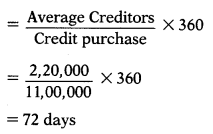Question 108.
N Ltd. gives the following information:
Current Ratio — 2.8
Total assets — ₹ 60,00,000
Fixed assets — ₹ 32,00,000
Current liabilities = ?
(A) ₹ 28,00,000
(B) ₹ 10,00,000
(C) ₹ 18,00,000
(D) ₹ 12,00,000
(B) ₹ 10,00,000
Current assets = 60,00,000 – 32,00,000 = 28,00,000
$$2.8=\frac{28,00,000}{x}$$
x = Current liabilities = 10,00,000

Question 109.
N Ltd. gives the following information:
Liquid ratio — 1.6
Current Assets — ₹ 28,00,000
Current Liabilities — ₹ 10,00,000
Stock = ?
(A) ₹ 28,00,000
(B) ₹ 10,00,000
(C) ₹ 18,00,000
(D) ₹ 12,00,000
(D) ₹ 12,00,000Question 110.
S Ltd. gives the following information:
Net working capital ₹ 2,80,000
Current ratio — 2.4
Liquid ratio — 1.6
Current Assets ?
(A) ₹ 2,00,000
(B) ₹ 2,80,000
(C) ₹ 4,80,000
(D) ₹ 3,60,000
(C) ₹ 4,80,000
Current Ratio = $$\frac{x}{y}$$
2.4 = $$\frac{x}{y}$$
2.4y = x
Working Capita] = CA – CL
2,80,000 = 2.4y – y
2,80,000 = 1.4y
y = 2,00,000
x = Current Assets = 2,00,000 × 2.4 = 4,80,000

Question 111.
Debtors as per working capital
statement = ₹ 3,00,000
Debtors collection period = 45 days
Gross profit ratio = 20%
Cash sales = ₹ 5,00,000
1 year = 365 days
Total sales = ?
(A) ₹ 24,00,000
(B) ₹ 19,00,000
(C) ₹ 29,00,000
(D) ₹ 25,00,000
(C) ₹ 29,00,000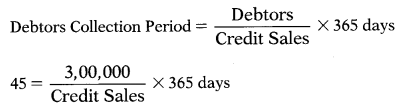Credit Sales = 24,00,000
Total sales = 24,00,000 + 5,00,000 = 29,00,000

Question 112.
Operating cycle days of Ramji Ltd. is 167 days. Other details are as follows:
Raw material stock velocity — 79 days
Debtors collection period — 70 days
WIP conversion period — 36 days
Finished goods conversion — 29 days period
Creditors payment period = ?
(A) 47 days
(B) 94 days
(C) 52 days
(D) 59 days
(A) 47 days
Let the creditors payment period be ‘x’.
79 + 70 + 36 + 29 – x = 167
– x = – 47
x = Creditors payment period = 47

Question 113.
Operating cost is ₹ 18,90,000.
Current assets Eire ₹ 5,20,000
Current liabilities are ₹ 1,00,000
Operating cycle days = ?
(Assume a 360 days year.)
(A) 80 days
(B) 99 days
(C) 19 days
(D) 70 days
(A) 80 days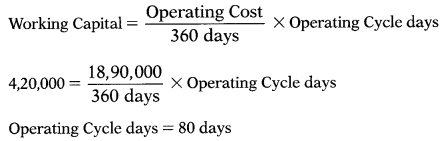Question 114.
NS Ltd. gives the following information:
Current Ratio = 2.4
Quick Ratio =1.0
Stock = ₹ 5,60,000
Current Assets = ?
(A) ₹ 9,60,000
(B) ₹ 6,90,000
(C) ₹ 4,00,000
(D) ₹ 4,60,000
(A) ₹ 9,60,000Question 115.
Gross profit ratio = 20%.
Stock velocity = 6 month
Gross profit for the year ended amounts to ₹ 5,00,000. Stock of the year is ₹ 20,000 more than what it was at the beginning of the year
Closing stock = ?
(A) ₹ 10,00,000
(B) ₹ 9,90,000
(C) ₹ 10,10,000
(D) ₹ 10,20,000
(C) ₹ 10,10,000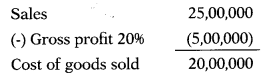Question 116.
No. of operating cycle in a year = 4.5
No. of days in year = 360 days
Working capital = 8,40,000
Operating cost = ?
(A) ₹ 35,00,000
(B) ₹ 37,80,000
(C) ₹ 36,40,000
(D) ₹ 38,80,000
(B) ₹ 37,80,000Question 117.
If current assets are ₹ 1,09,05,750 and current liabilities are ₹ 32,50,000 then maximum permissible bank finance as per first method of Tandon Committee norms is –
(A) ₹ 57,41,813
(B) ₹ 49,29,313
(C) ₹ 52,29,813
(D) ₹ 49,41,813
(A) ₹ 57,41,813
Maximum permissible bank finance as per Tandon Committee norms:
Method 1: 75% of (Current Assets – Current Liabilities)
= 0.75 × (1,09,05,750 – 32,50,000)
= 57,41,813

Question 118.
Debtors velocity = 3 months
Sales = ₹ 25,00,000
Bills receivable & Bills payable were ₹ 60,000 and ₹ 36,667 respectively.
Sundry debtors = ?
(A) ₹ 6,25,000
(B) ₹ 5,25,000
(C) ₹ 5,65,000
(D) ₹ 6,65,000
(C) ₹ 5,65,000x = Account Receivable = 6,25,000
Debtors + Bills Receivable = Account Receivable
x + 60,000 = 6,25,000
x = Debtors = 5,65,000

Question 119.
Creditors velocity = 2 months.
Cost of goods sold = ₹ 20,00,000
Opening stock = ₹ 9,90,000
Closing stock = ₹ 10,10,000
Bills receivable & Bills payable were ₹ 60,000 and ₹ 36,667 respectively.
Creditors = ?
(A) 3,36,667
(B) 3,66,333
(C) 3,30,367
(D) 3,00,000
(D) 3,00,000
Opening stock + Purchase – Closing stock = Cost of goods sold
9,90,000 + x – 10,10,000 = 20,00,000
x Purchase = 20,20,000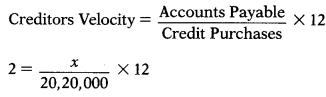x = Accounts Payable = 3,36,667
Creditors + Bifis Payable Account Payable
x + 36,667 = 3,36,667
x = Creditors = 3,00,000

Question 120.
If current assets are ₹ 1,09,05,750 and current liabilities are ₹ 32,50,000 then maximum permissible bank finance as per second method of Tandon Committee norms is –
(A) ₹ 57,41,813
(B) ₹ 49,29,313
(C) ₹ 52,29,813
(D) ₹ 49,41,813
(B) ₹ 49,29,313
Maximum permissible bank finance as per Tandon Committee norms:
Method 2: 75% of Current Assets – Current Liabilities = (0.75 × 1,09,05,750) – 32,50,000
= 49,29,313

Question 121.
When the current ratio is 2:5, and the amount of current liabilities is ₹ 25,000, what is the amount of current assets?
(A) ₹ 62,500
(B) ₹ 12,500
(C) ₹ 10,000
(D) None of these
(C) ₹ 10,000

Question 122.
Total ‘cost of sales’ and ‘sales’ of Gama Ltd. is ₹ 3,19,80,000 and ₹ 4,13,40,000 respectively. It makes 20% sales on cash basis. Debtors are allowed 2 months credit period. If company calculates working capital on cash cost basis then debtors are –
(A) ₹ 55,12,000
(B) ₹ 42,12,000
(C) ₹ 42,64,000
(D) ₹ 55,64,000
(C) ₹ 42,64,000Question 123.
If credit sales for the year is ? 5,40,000 and Debtors at the end of year is ? 90,000 the Average Collection Period will be?
Note: 1 year = 365 days
(A) 30 days
(B) 61 days
(C) 90 days
(D) 120 days
(B) 61 days

Question 124.
Total wages for the year of Rakshit Ltd., a listed company are ₹ 64,80,000 out of which ₹ 2,40,000 are paid in cash immediately after they became due. Lag in payment of wages – 1 month. If you are appointed to prepare working capital statement for the company, then how much outstanding wages you will take while preparing working capital statement?
(A) ₹ 5,40,000
(B) ₹ 5,50,000
(C) Not enough data
(D) ₹ 5,20,000
(D) ₹ 5,20,000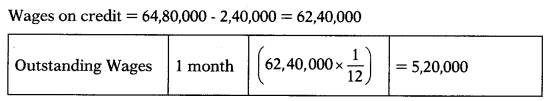Question 125.
K Ltd. had sales last year of ₹ 26,50,000, including cash sales of ₹ 2,50,000. If its average collection period was 36 days, its ending accounts receivable balance is closest to (Assume a 365 day year.)
(A) ₹ 2,40,000
(B) ₹ 2,36,712
(C) ₹ 2,63,127
(D) ₹ 2,40,721
(A) ₹ 2,40,000

Question 126.
Outstanding overheads appearing in balance sheet are 9,75,000. Lag in payment g of overheads is 30 days. Overheads accrue evenly throughout the year, total overheads incurred by the company are –
(A) ₹ 1,17,00,000
(B) ₹ 32,500
(C) ₹ 2,92,50,000
(D) ₹ 1,92,50,000
(A) ₹ 1,17,00,000
Outstanding Overheads = Total Overheads = $$\times \frac{\text { Lag in payment of overheads }}{360}$$
9,75000 = Total Overheads × $$\frac{30}{360}$$

Question 127.
From the information given below calculate the amount of fixed assets. Fixed assets to proprietors fund = 1.25, Net Working Capital = ₹ 6,00,000.
(A) 20,00,000
(B) 24,00,000
(C) 3,42,857
(D) 8,00,000
(B) 24,00,000

Question 128.
If a firm has ₹ 100 in inventories, a current ratio equal to 1.2, and a quick ratio equal to 1.1, what is the firm’s Net Working Capital?
(A) ₹ 10
(B) ₹ 100
(C) ₹ 200
(D) ₹ 1,200
(C) ₹ 200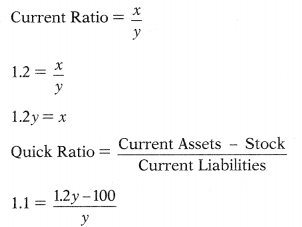y = 1000
x = Current Assets 1,000 × 1.2= 1,200
Working Capital 1,200 – 1,000 200

Question 129.
A company plans to manufacture and sell 400 units of domestic appliances per month at a price of ₹ 600 each. The ratios of cost to selling price are –
Raw materials — 30%
Packing material — 10%
Direct labour — 15%
Direct expenses — 5%
Fixed overhead are ₹ 4,32,000 p.a.
Finished goods stock = 200 units
Working days in year are taken as 300 days for budget year. Norms maintained for work-in-progress is 7 days. Finished goods stock and WIP stock will appear in balance sheet and working capital of the company at –
(A) ₹ 31,920;₹ 80,000
(B) ₹ 80,000;₹ 31,920
(C) ₹ 90,000; ₹ 67,200
(D) ₹ 90,000;₹ 31,920
(D) ₹ 90,000;₹ 31,920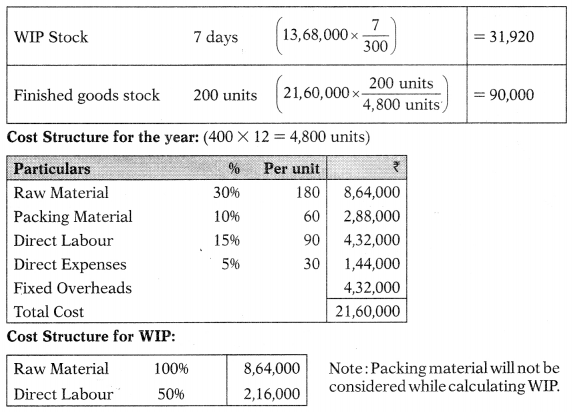Question 130.
Following is the balance sheet of PBX Ltd. Calculate maximum permissible bank finance by Third Method of Tandon Committee norms. Assume the level of Core Current Assets to be ₹ 60 lakhs.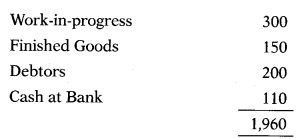(A) 450 1akhs
(B) 360 lakhs
(C) 315 Iakhs
(D) 425 lakhs
(C) 315 Iakhs
Current Assets = 960 Lakhs; Current Liabilities = 360 Lakhs
Maximum permissible bank borrowing as per 3rd method of Tandon Committee norms:
= [75% of (Current Assets – Core Current Assets)] – Current Liabilities
= [0.75 × (960 – 60)] – 360
= 315 Lakhs

Question 131.
Current assets & current liabilities of Deelip Ltd. are 9,60,000 and 3,60,000 respectively. Maximum permissible bank finance as per Tandon Committee norms is 3,15,000. What are the core current assets of Deelip Ltd?
(A) ₹ 60,000
(B) ₹ 45,000
(C) ₹ 30,000
(D) ₹ 90,000
(A) ₹ 60,000
3,15,000 = [75% of (Current Assets- Core Current Assets)] – Current Liabilities
3,15,000 = [0.75 × (9,60,000 – x)] – 3,60,000
3,15,000 = 7,20,000 – 0.75x – 3,60,000
45,000 = – 0.75x
x = Core Current Assets = 60,000

Question 132.
Maximum permissible bank finance as per 1st method of Tandon committee norms is ₹ 3,18,75,000. Long terms loans are ₹ 5,58,80,450. Current liabilities are ₹ 70,00,000. Current assets of the company are –
(A) ₹ 4,25,00,000
(B) ₹ 4,95,00,000
(C) ₹ 3,55,00,000
(D) ₹ 1,10,00,726
(B) ₹ 4,95,00,000
3,18,75,000 = 75% of (Current Assets – Current Liabilities)
3,18,75,000 = 0.75 × (x – 70,00,000)
3,18,75,000 = O,75x – 52,50,000
3,71,25,000 = 0.75x
x Current Assets = 4,95,00,000

Question 133.
From the following information calculate the responsiveness of ROCE for changes in current assets ie. Working Capital Leverage.Current assets are likely to decline by 20%over the existing level
(A) 0.405;0.435
(B) 0.403;0.453
(C) 0.453; 0.404
(D) 0.435; 0.405
(D) 0.435; 0.405Question 134.
MNO Ltd. submit following figures: Current Assets = ₹ 232.5 lakh
Core current assets = ₹ 30 lakh
Maximum permissible bank finance as third method of Tandon Committee norms = ₹ 55.625 lakh
Working capital in this case is –
(A) ₹ 136.25
(B) ₹ 106.05
(C) ₹ 163.25
(D) ₹ 160.05
(A) ₹ 136.25
55.625 = [75% of (Current Assets – Core Current Assets)] – Current Liabilities
55.625 = [0.75 × (232.5 – 30)] – x
55.625 = 151.875 – x
x = Current Liabilities 96.25
Working Capital = 232.5 – 96.25 = 136.25

Question 135.
Management of Royal Ltd. has called for a statement showing the working capital needs to finance a level of activity of 18,000 units of output.Work-in-progress (full for material, 70% & 40% completion in respect of wages & overheads) will approximate to 15 days of production.
1 year = 360 days. Calculate closing stock of WIP.
(A) ₹ 22,125
(B) ₹ 20,652
(C) ₹ 20,562
(D) ₹ 20,875
(A) ₹ 22,125
Cost Structure for WIP: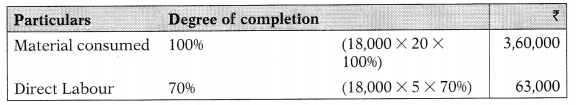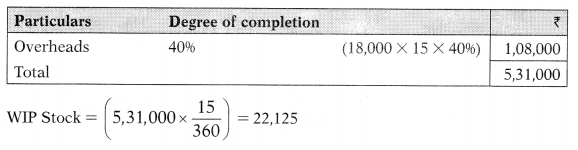Question 136.
Management of Royal King Ltd. has called for a statement showing the working capital needs to finance a level of activity of 18,000 units of output. Cost structure per unit: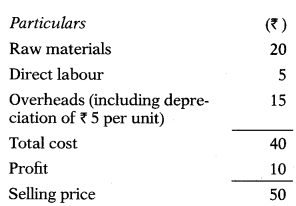Work-in-progress (full for material, 70% & 40% completion in respect of wages & overheads) will approximate to 15 days of production. 1 year = 360 days. Calculate closing stock of WIP on cash cost basis.
(A) ₹ 22,125
(B) ₹ 19,250
(C) ₹ 19,215
(D) ₹ 20,625
(D) ₹ 20,625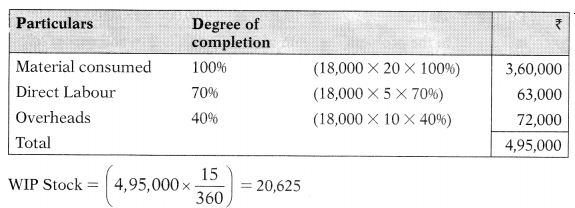Question 137.
Opening and closing stock of SVK Ltd. is ₹ 1,20,000 & ₹ 1,80,000 respectively. Material consumed in income statement is shown at ₹ 3,60,000. Suppliers of material extend 4 week credit. How much of creditors will be shown in preparation of working capital statement?
(A) ₹ 32,380
(B) ₹ 32,830
(C) ₹ 32,308
(D) ₹ 32,803
(C) ₹ 32,308
Opening Raw Material + Purchases – Closing Raw Material = Material Consumed
1,20,000 + Purchases – 1,80,000 = 3,60,000
Purchases = 4,20,000
Creditors 4 weeks $$\left(4,20,000 \times \frac{4}{52}\right)$$ = 32308

Question 138.
Opening and closing stock of SVK Ltd. is ₹ 1,20,000 & ₹ 1,80,000 respectively. Material consumed in income statement is shown at ₹ 3,60,000. Suppliers of material extend 4 week credit. How much of creditors will be shown in preparation of working capital statement if cash purchase is ₹ 50,000?
(A) ₹ 28,246
(B) ₹ 28,642
(C) ₹ 28,426
(D) ₹ 28,462
(D) ₹ 28,462
Opening Raw Material + Purchases- Closing Raw Material = Material Consumed
1,20,000 + Purchases – 1,80,000 = 3,60.000
Purchases = 4,20,000
Credit purchase = 4,20,000 – 50,000 = 3,70,000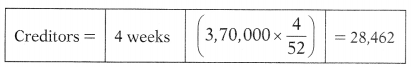Question 139.
The following cost information per unit has been ascertained for annual production of 36,000 units:
Variable manufacturing overheads = ₹ 2 Fixed manufacturing overheads = ₹ 1 Lag in payment of overheads = one month
Expected production and sales are 48,000 units and 35,000 units respectively.
Outstanding overheads will be shown in working capital statement at –
(A) ₹ 11,000
(B) ₹ 11,667
(C) ₹ 12,000
(D) ₹ 12,500
(A) ₹ 11,000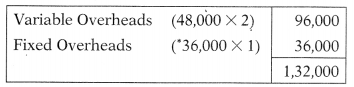Fixed overheads are × 1 per unit at current level of activity i..e. 36,000 packets.
Hence total fixed overheads at current level of activity 36,000 × 1 = 36,000. Fixed
overheads remains same hence even at increased production of 48,000 packets
fixed overheads will remain same i..e. ₹ 36,000.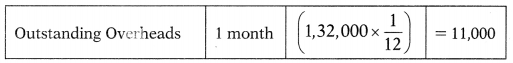Question 140.
Calculate the debtors on cash cost basis form the following information for working capital purpose: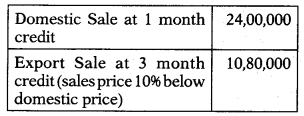Rate of gross profit is 20%.
(A) ₹ 4,70,000
(B) ₹ 4,00,000
(C) ₹ 3,76,000
(D) ₹ 4,76,000
(B) ₹ 4,00,000
Calculation of cost of goods sold: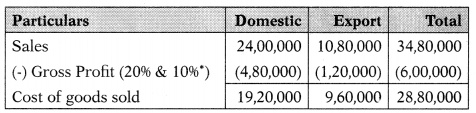It is stated in the problem that rate of gross profit is 2096 and export sales price 1096 below domestic price. Rate of gross profit for export sale has to be taken 10%. Further export sale has to be made 10096 from 90% for the purpose of calculation of gross profit.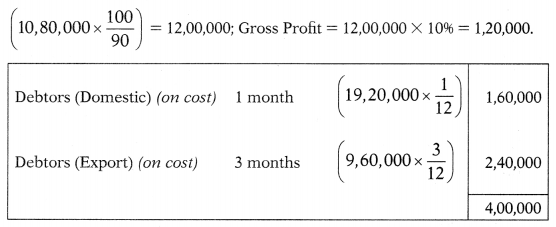Question 141.
From the following information calculate the working capital:
Equity share capital — 18,00,000
Stock — 3,15,000
Income tax payable — 56,250
Outstanding expenses — 1,40,000
Prepaid expenses — 37,500
Debtors — 4,00,000
Creditors — 1,50,000
The management is of the opinion to make 12% margin for contingencies on computed figure.
(A) ₹ 4,81,250
(B) ₹ 4,59,370
(C) ₹ 4,87,500
(D) ₹ 4,51,370
(C) ₹ 4,87,500Question 142.
From the following information calculate the net working capital:
Current assets — 92,75,000
Current liabilities — 34,62,500
The management is of the opinion to make 20% margin for contingencies on net working capital.
(A) ₹ 69,75,000
(B) ₹ 69,65,625
(C) ₹ 75,75,000
(D) ₹ 72,65,625
(D) ₹ 72,65,625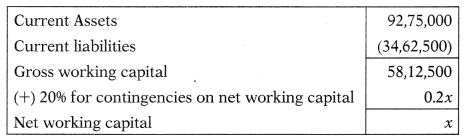58,12,500 + 0.2.x = x
58,12,500 = 0.8x
x = Net working capital = 72,65,625

Question 143.
The CTC Ltd. is attempting to establish a current assets policy. Fixed assets are ₹ 6,00,000 and the firm plans to maintain a 50% debt-to-assets ratio. The interest rate is 10% on all debts. Three alternative current assets policies are under consideration 40%, 50% and 60% of projected sales. Company expects to earn 15% before interest and taxes on sales of ₹ 30,00,000. The effective tax rate is 40%. What is the expected return on equity if company follows ‘AggressivePolicy’?
(A) 19.71%
(B) 24.00%
(C) 16.50%
(D) 18.67%
(B) 24.00%Question 144.
The CTC Ltd. is attempting to establish a current assets policy. Fixed assets are ₹ 6,00,000 and the firm plans to maintain a 50% debt-to-assets ratio. The interest rate is 10% on all debts. Three alternative current assets policies are under consideration 40%, 50% and 60% of projected sales. Company expects to earn 15% before interest and taxes on sales of ₹ 30,00,000. The effective tax rate is 40%. What is the expected return on equity if company follows ‘Moderate Policy?
(A) 19.71%
(B) 24.00%
(C) 16.50%
(D) 18.67%
(A) 19.71%Question 145.
The CTC Ltd. is attempting to establish a current assets policy. Fixed assets are ₹ 6,00,000 and the firm plans to maintain a 50% debt-to-assets ratio. The interest rate is 10% on all debts. Three alternative current assets policies are under consideration – 40%, 50% and 60% of projected sales. Company expects to earn 15% before interest and taxes on sales of ₹ 30,00,000. The effective tax rate is 40%. What is the expected return on equity if company follows ‘ModeratePolicy}
(A) 19.71%
(B) 24.00%
(C) 16.50%
(D) 18.67%
(C) 16.50%Question 146.
Strong Cement Ltd. has an installed capacity of producing 1.25 lakh tones of cement per annum; its present capacity utilization is 80%. The major raw material to manufacture cement is limestone which is obtained on cash basis from a company located near the plant. The company produces cement in200 kg drum. Calculate the number of drums to be manufactured.
(A) 50,000 drums
(B) 60,000 drums
(C) 80,000 drums
(D) 1,00,000 drums
(A) 50,000 drums
Production in tone = 1,25,000 tone X 80% = 1,00,000
Number of drums = $$\frac{1,00,000 \text { tone }}{200 \mathrm{~kg} \text { i.e. } 0.2 \text { tone }}$$= 5,00,000 Drums

Question 147.
M Ltd. is engaged in large scale customer retailing. From the following information, you are required to forecast its working capital requirement:
Projected annual sales — ₹ 65 lakhs
Net profit on cost of sales — 20%
Credit allowed to debtors — 10 weeks
Credit allowed by creditors — 4 weeks
Stock carrying (in terms of 8 weeks cost of sales requirement)
Add 10% company calculates debtors on sales to allow for contingencies.
(A) ₹ 14,33,333
(B) ₹ 15,66,667
(C) ₹ 16,33,333
(D) ₹ 16,66,667
(D) ₹ 16,66,667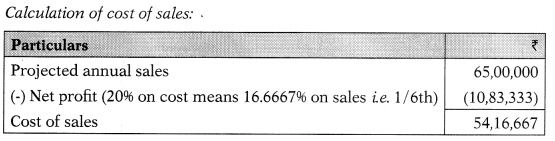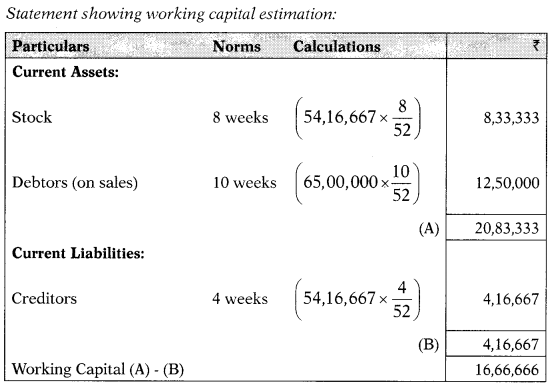Question 148.
A company has prepared its annual budget, relevant details of which are reproduced below:
Sales ₹ 46.80 lakh (25% cash sales and balance on credit): 78,000 units
Labour cost: ₹ 6 per unit
Wages are paid as follows:
For 1st & 2nd week: in the 3rd week
For 3rd & 4th week: in the next week.
Assume 1 year = 52 weeks.
How much outstanding wages will appear × in working capital statement?
(A) ₹ 18,000
(B) ₹ 20,000
(C) ₹ 16,000
(D) ₹ 22,000
(A) ₹ 18,000
Total wages = 78,000 × 6 = 4,68,000
Wages are paid for 1st and 2nd week in the 3rd week & for 3rd and 4th week in the next week. Thus, wages are paid 3rd & 5th week which shows that lag in payment of wages is 2 week.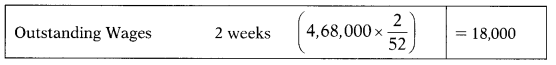Question 149.
Following details are available for Pratik Ltd.
Maximum permissible bank finance as per second method of Tandon Committee norms: ₹ 11,96,188
Current liabilities: ₹ 3,73,750
What will be the amount of maximum permissible bank finance as per first method of Tandon Committee Norms?
(A) ₹ 12,98,625
(B) ₹ 12,89,265
(C) ₹ 12,89,625
(D) ₹ 12,98,265
(C) ₹ 12,89,625
11,96,188 = 75% of Current Assets – Current Liabilities
11,96,188 = 0.75 × x – 3,73,750
11,96,188 = 0.75x -3,73,750
x = Current Assets = 20,93,250
Method 1: 75% of (Current Assets – Current Liabilities)
= 75% × (20,93,250 – 3,73,750)
= 12,89,625

Question 150.
Selling price is ₹ 50 per unit and production and sales for the year are 69,000 units and 75,000 units respectively. Direct wages are 10% of selling price. There is regular production and sales cycle, and wages and overheads accrue evenly. Wages & overheads are paid in the next month of accrual. One production process cycle is completed in two month. How much outstanding wages will be shown in working capital statement?
(A) ₹ 28,750
(B) ₹ 57,500
(C) ₹ 31,250
(D) ₹ 28,250